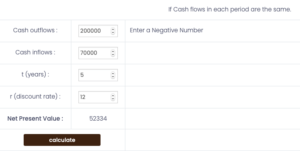# Description

Net Present Value (NPV) illustrates the difference between the present value of cash inflows and the present value of cash outflows over a given period of time. NPV is often incorporated in investment planning and capital budgeting to analyse the profitability of a project.

Net Present Value Formula: Cashflow/(1+r)^n – Initial Investment

Here:

n= number of periods between now and the moment when we will receive money

r= discount rate (interest rate used in cash flow analysis)

The NPV formula may vary depending on the number and consistency of future cash flows. Moreover, if the NPV of an investment or project is positive, the discounted present value of all future cash flows can be found attractive. However, if the NPV comes out to be negative, then the investment or project should not be accepted. There is also a chance that cash inflows’ present value equals the present value of cash outflows. In this circumstance, it is up to the investor to accept or reject the investment opportunity or a project.

## Calculation of Net Present Value

Let’s suppose an industry is looking to expand in the near future. The industry has two options: either to invest where it generates the same cash flow in each period or to invest where it receives varying cash flow in each period.

The industry is looking forward to investing \$200,000 as an initial investment, and the expected discount rate is 12% annually. Taking option 1, the cash flows are \$70000 for 5 years. We would get:The Net Present Value for the same cash flow in each period is \$52,334.

Now, taking option 2 into consideration, assuming that the Cash Flow for year 1 is \$60,000, for year 2 \$72,000, for year 3 \$88,000, for year 4 \$59,000, and year 5 \$77,500.

We would get:The Net Present Value for different cash flows in each period is \$55,077.

In these two options, where both NPVs are positive, the investor would consider opting for the option with the highest NPV figure. In this scenario, the investor would want to opt for option 2, where the NPV figure is \$55,077 greater than option 1 NPV figure of \$52,334.